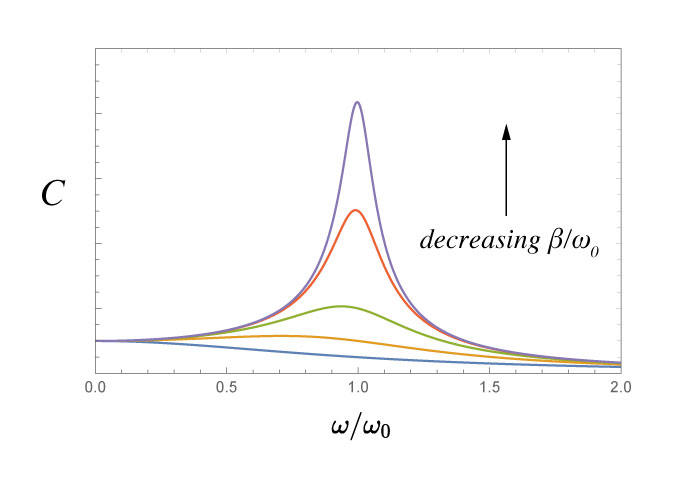Restoring Forces

\begin{equation} \Sigma F = - kx = m \ddot x \label{eq:hookeslawsumsofforces} \end{equation}

The general solution for a linear restoring force: \begin{equation} x(t) = A \cos \left(\omega t \right) + B \sin \left( \omega t \right) \end{equation} or, if we use the phase constant: $\phi$ \begin{equation} x(t) = C \cos \left(\omega t + \phi \right) \end{equation}

Detailed Solution of Hooke's Law

We'd like to actually solve the diff eq, rather than just 'guessing' at a solution. Here is one method:

Start with the Sums of Forces equation: \begin{equation} m \frac{dv}{dt} = - k x \label{eq:hookeslaw} \end{equation}

Another route:

Rewriting Hooke's Law as \begin{equation} \frac{d^2 x}{dt^2} + \omega^2 x = 0 \end{equation} we could attempt to solve it by assuming that the solution must take the form: $$x(t) = e^{qt}$$, and then do some work to figure out what $q$ is. (we'll save that...)

Analogous systems

Simple Pendulum

For small angles, the tangential force on the pendulum bob will be given by: $$F_\textrm{tang} = -mg \sin \theta$$

When $\theta$ is very small ($\theta < .2$ radians), $\sin \theta \approx \theta$. Using the fact that $s = L \theta$, we can also write: $$F_\textrm{tang} = -\frac{mg}{L}{s}$$

This is essentially a restoring force, just like we had for the mass/spring system. $$F = -kx \;\;\Rightarrow \;\; F = -\frac{mg}{L}s$$

Other coordinates?

A slightly better coordinate to describe the motion of the pendulum might be $\theta$. That would be a slightly more general coordinate system.

\begin{equation} \ddot{\theta} + \frac{g}{l}\theta = 0 \end{equation}

Damping

$$F = m \ddot{x} = -kx - b \dot{x}$$

Clean it up:

\begin{equation} \ddot{x} + 2 \beta \dot{x} + w_0^2 x = 0 \label{eq:dampedoscillatoreqofmotion} \end{equation} where $\beta \equiv \frac{b}{2m}$ and $\omega_0 \equiv \sqrt{\frac{k}{m}}$

A general solution would look like: $$x \propto e^{\alpha t}$$

\begin{equation} \alpha = -\beta \pm \sqrt{\beta^2 - \omega_0} = 0 \end{equation}

There are 3 possibilities now:

1. Overdamped: $\beta \gt \omega_0$
2. Critically Damped: $\beta = \omega_0$
3. Underdamped: $\beta \lt \omega_0$

Overdamped

If $$\beta \gt \omega_0$$ then the exponent $\alpha$ in our solution $x = e^{\alpha t}$ will be negative and real.

Critically Damped

If $$\beta = \omega_0$$ then solution becomes $x(t) = \left(A + A' t \right) e^{-\beta t}$

Underdamped

If $$\beta < \omega_0$$ then we see that $\sqrt{\beta^2 - \omega^2}$ will be imaginary.

Plotted here are the 3 different regimes of a damped oscillator.

Damped and Driven

$$F = m \ddot{x} = -kx - b \dot{x} + F_0 \sin \left(\omega t \right)$$

So, now we can solve this: $$m \ddot{x} + kx + b \dot{x} = F_0 \sin \left(\omega t \right)$$ We want our solution to be the sum of the characteristic and particular solutions: $$x(t) = x_c(t) + x_p(t)$$ that is, a general solution for the homogeneous part, and a particular solution for the inhomogeneous equation.The response of the damed-driven oscillator (bottom) is shown to be the sum of the transient term (top) and the steady state (middle) components.

It's helpful to imagine the combination of the two terms in a graphical form.

Resonance

Here we see the change in the $C$ value as a function of drive frequency (in relation to resonant frequency: $\frac{\omega}{\omega_0}$).

Maximize $C(\omega)$ to find the driving frequency $\omega$ that will lead to the largest response.

$$C = \frac{f_0}{\sqrt{\left(\omega_0^2 - \omega^2 \right)^2 + 4 \beta^2 \omega^2}}$$If we decrease the damping (and keep $\omega_0$ constant), we can see the peak of the resonance increasing.

This graph shows 5 different damped driven oscillators. The differences in each curve is the ratio of the damping constant to the resonant frequency. Notice the change in shape.

Quality Factor

The Quality Factor is the ratio of the resonant frequency, $\omega_0$, and the damping $\beta$.